# EDA for High-Throughput Data

Lucas Schiffer
August 19, 2016

Data Analysis for the Life Sciences

### Today's Topics

• Introduction
• Volcano Plots
• p-value Histograms
• Data Boxplots and Histograms
• MA plot
• Exercises

### Introduction

• How is EDA for high-throughput data different?

“If Edison had a needle to find in a haystack, he would proceed at once with the diligence of the bee to examine straw after straw until he found the object of his search… I was a sorry witness of such doings, knowing that a little theory and calculation would have saved him ninety per cent of his labor.” - Nikola Tesla

### Volcano Plotshttps://tinyurl.com/zxr4s8u

• Plot of -log(p-values) vs. effect size
• The two quantities should be inversely proportional

### Volcano Plots

# gene expression p-values
data(GSE5859Subset)
g <- factor(sampleInfo$group) results <- rowttests(geneExpression,g) pvals <- results$p.value

# nullified p-values
m <- nrow(geneExpression)
n <- ncol(geneExpression)
randomData <- matrix(rnorm(n*m), m, n)
nullpvals <- rowttests(randomData, g)$p.value  ### Volcano Plots plot(results$dm, -log10(results$p.value), xlab = "Effect Size", ylab = "-log(p-values)")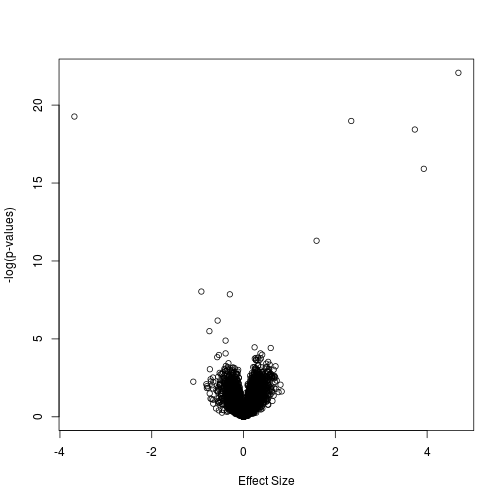### p-value Histograms hist(nullpvals, ylim = c(0, 1400))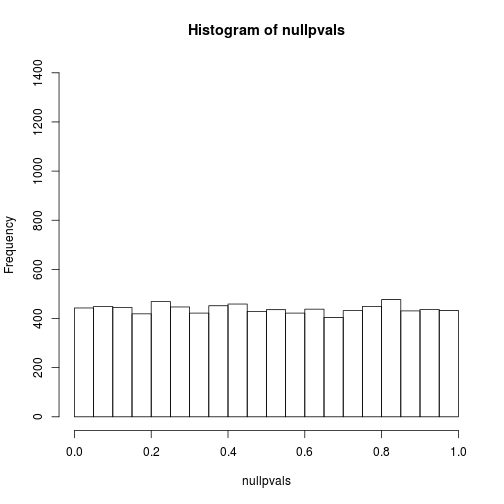hist(pvals, ylim = c(0, 1400))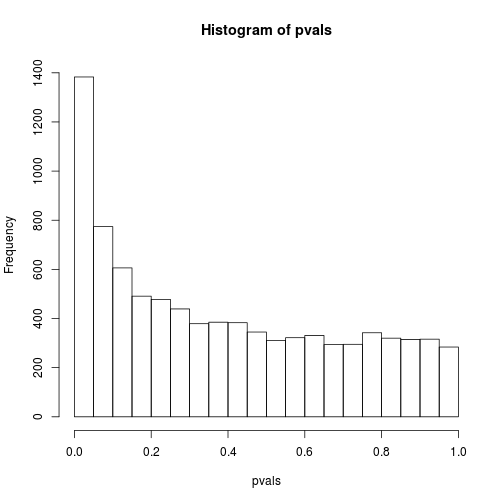### p-value Histograms • When an expected null result looks like a alternative? • Samples may be correlated, but can test for this • Permute the samples, plot of p-values should be normal ### p-value Histograms permg <- sample(g) permresults <- rowttests(geneExpression, permg) hist(permresults$p.value)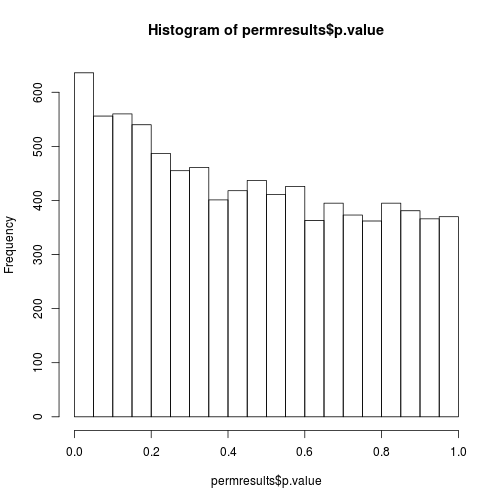### Data Boxplots and Histograms

data(GSE5859)
ge <- exprs(e) ##ge for gene expression
ge[, 49] <- ge[, 49]/log2(exp(1)) ##error
boxplot(ge, range = 0, names = 1:ncol(e), col = ifelse(1:ncol(ge) == 49, 1, 2))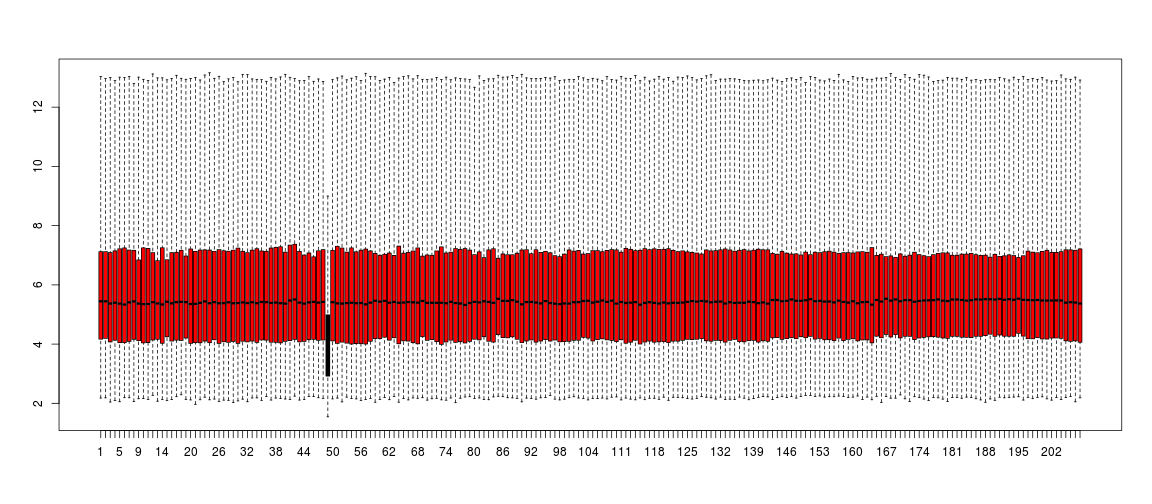### No boxes, no problem… kaboxplot!

qs <- t(apply(ge, 2, quantile, prob=c(0.05, 0.25, 0.5, 0.75, 0.95)))
matplot(qs,type="l",lty=1)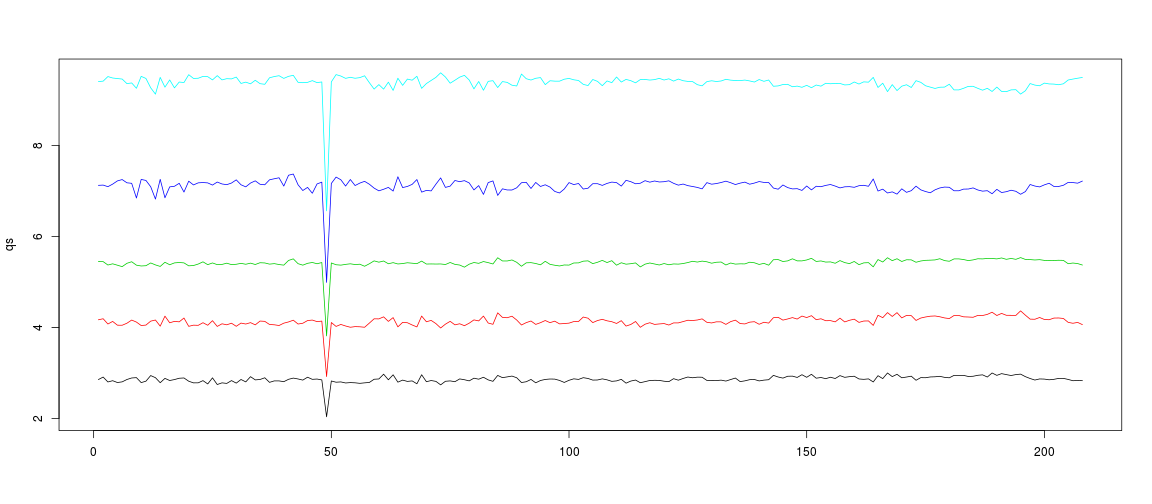### Say shistogram 5 times fast!

shist(ge, unit = 0.5)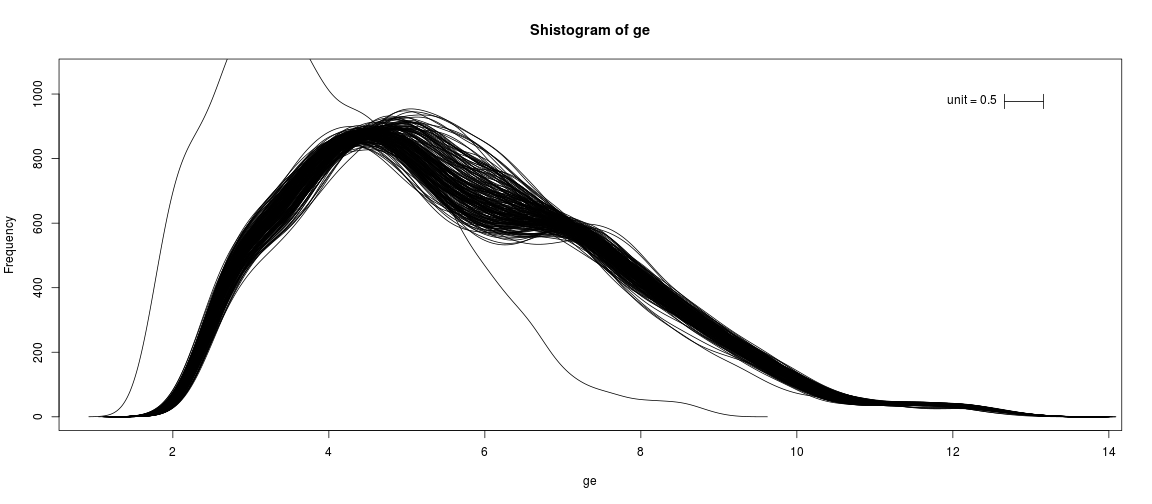### MA plotMicroarray platforms https://tinyurl.com/htv3de8

• Scatterplots don't work very well
• Plot of (log(red) - log(green)) vs. average of log(red) and log(green)
• Known as MA plot because it is a Minus vs. Average of the log intensities

### MA plot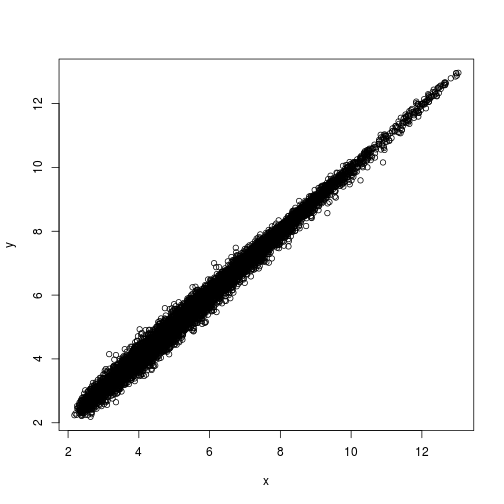• Approximate sd(y-x) ?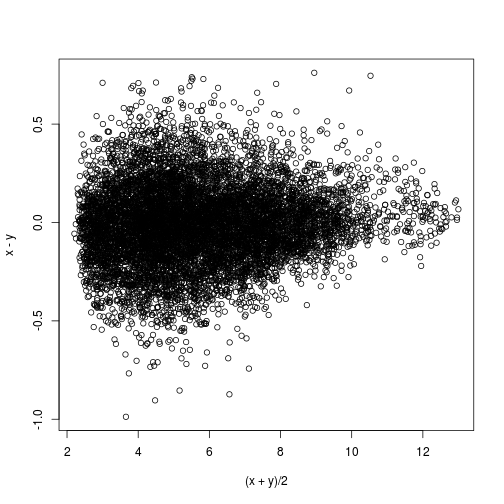sd(y-x)

 0.2025465